# pymaid.sparseness¶

pymaid.sparseness(x, which='LTS')[source]

Calculate sparseness.

Sparseness comes in two flavors:

Lifetime kurtosis (LTK) quantifies the widths of tuning curves (according to Muench & Galizia, 2016):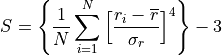where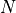is the number of observations,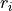the value of observation, and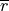and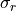the mean and the standard deviation of the observations’ values, respectively. LTK is assuming a normal, or at least symmetric distribution.

Lifetime sparseness (LTS) quantifies selectivity (Bhandawat et al., 2007):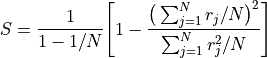whereis the number of observations, and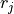is the value of an observation.

Notes

NaN values will be ignored. You can use that to e.g. ignore zero values in a large connectivity matrix by changing these values to NaN before passing it to pymaid.sparseness.

Parameters
• x (DataFrame | array-like) – (N, M) dataset with N (rows) observations for M (columns) neurons. One-dimensional data will be converted to two dimensions (N rows, 1 column).

• which ("LTS" | "LTK") – Determines whether lifetime sparseness (LTS) or lifetime kurtosis (LTK) is returned.

Returns

pandas.Series if input was pandas DataFrame, else numpy.array.

Return type

sparseness

Examples

Calculate sparseness of olfactory inputs to group of neurons:

>>> import numpy as np
>>> import matplotlib.pyplot as plt
>>> # Generate adjacency matrix